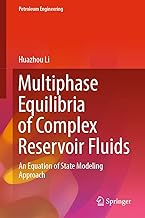• library@msu.ac.th
• Academic Resource Center Mahasarakham University
• library@msu.ac.th
• Academic Resource Center Mahasarakham Universityหมวดหมู่### Multiphase Equilibria of Complex Reservoir Fluids

"This short monograph focuses on the theoretical backgrounds and practical implementations concerning the thermodynamic modeling of multiphase equilibria of complex reservoir fluids using cubic equations of state. It aims to address the increasing needs of multiphase equilibrium calculations that arise in the compositional modeling of multiphase flow in reservoirs and wellbores. It provides a state-of-the-art coverage on the recent improvements of cubic equations of state. Considering that stability test and flash calculation are two basic tasks involved in any multiphase equilibrium calculations, it elaborates on the rigorous mathematical frameworks dedicated to stability test and flash calculation. A special treatment is given to the new algorithms that are recently developed to perform robust and efficient three-phase equilibrium calculations. This monograph will be of value to graduate students who conduct research in the field of phase behavior, as well as software engineers who work on the development of multiphase equilibrium calculation algorithms. "

ใส่ตะกร้า
• ISBN9783030874391
• ประเภท E-Book
• ผู้แต่ง Huazhou Li
• สำนักพิมพ์ Springer International Publishing
• ครั้งที่พิมพ์ 1
• ปีที่พิมพ์2022
• ภาษาภาษาอังกฤษ
• หมวดหมู่สิ่งแวดล้อม
###### : ข้อมูลหนังสือ

"This short monograph focuses on the theoretical backgrounds and practical implementations concerning the thermodynamic modeling of multiphase equilibria of complex reservoir fluids using cubic equations of state. It aims to address the increasing needs of multiphase equilibrium calculations that arise in the compositional modeling of multiphase flow in reservoirs and wellbores. It provides a state-of-the-art coverage on the recent improvements of cubic equations of state. Considering that stability test and flash calculation are two basic tasks involved in any multiphase equilibrium calculations, it elaborates on the rigorous mathematical frameworks dedicated to stability test and flash calculation. A special treatment is given to the new algorithms that are recently developed to perform robust and efficient three-phase equilibrium calculations. This monograph will be of value to graduate students who conduct research in the field of phase behavior, as well as software engineers who work on the development of multiphase equilibrium calculation algorithms. "